# Problems of the Week

Contribute a problem

# 2017-12-11 Basic

The four open containers shown below are all filled to the same height with water. Which container has the lowest pressure on the base of the container exerted by the water?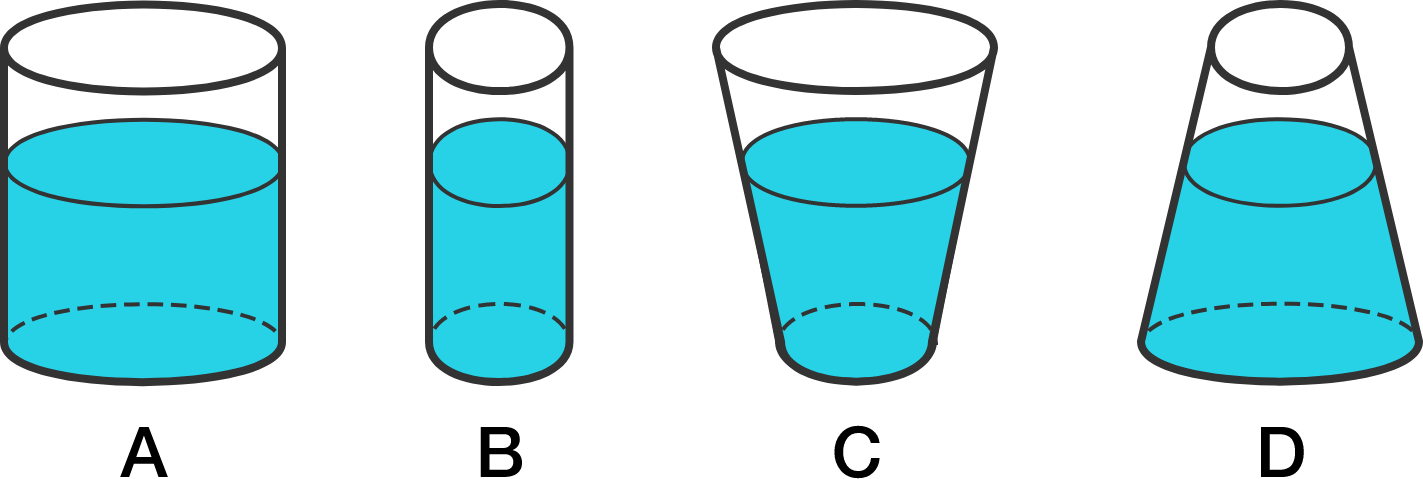Among 5 dogs, there are exactly 4 named Jeff. They all speak, in the order shown: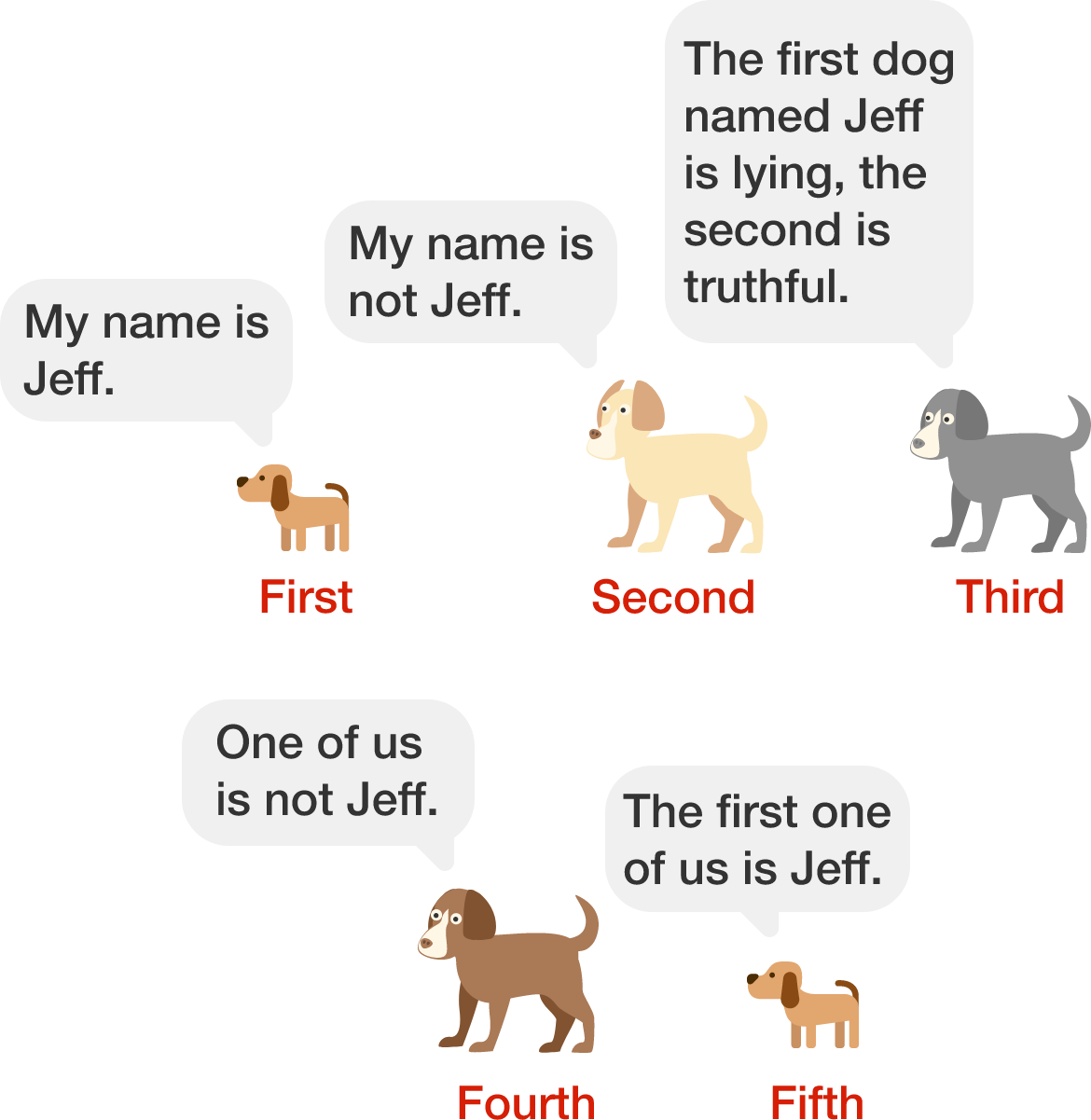If exactly one dog is lying, and all of the dogs are aware of who is named Jeff and who is the liar, then which dog is lying?

Suppose you want to fill a $150\times60\times37$ cuboid with $5 \times 5 \times 5$ cubes. To calculate how many fit, you divide the two volumes:

$\dfrac{37\times60\times150}{5\times5\times5} = 2664.$

You conclude that given optimal placement, 2664 of the cubes fit inside the cuboid. Is this correct?

Assume that all the faces of the cubes are in parallel with the faces of the cuboid.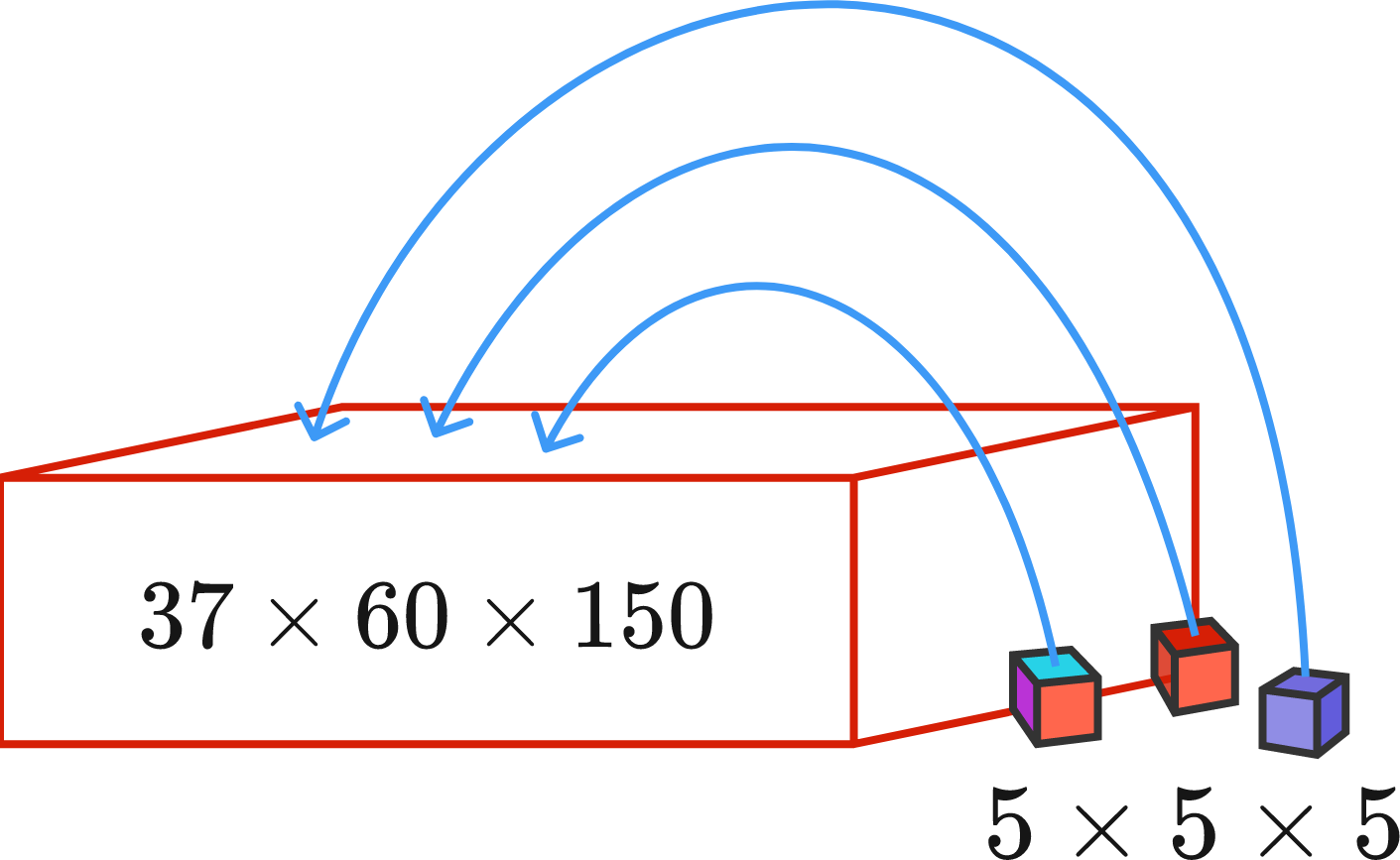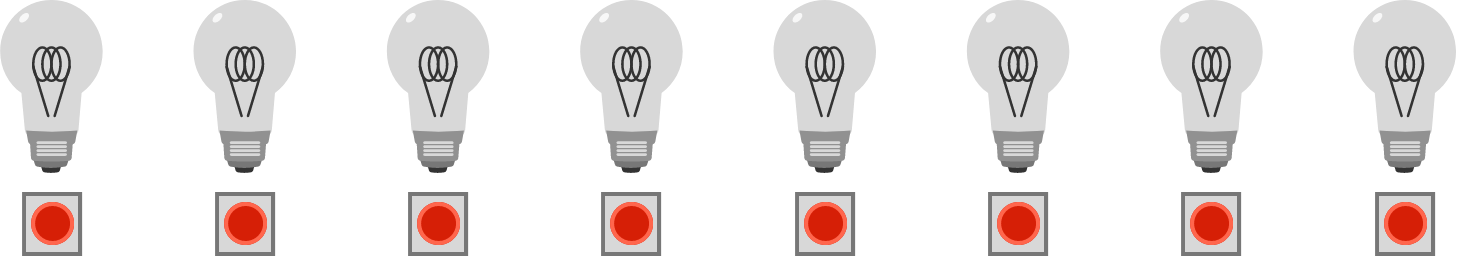As shown above, I have a row of 8 light bulbs, initially all switched off. There is a button below each light bulb that will toggle the bulb on or off.

I want them to be in an alternating off-on state, as shown below.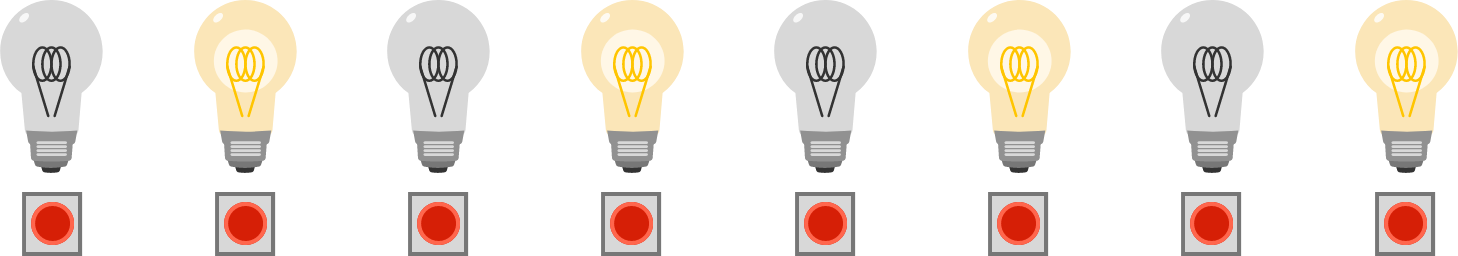To do so, I press a block of consecutive buttons in a row. Here is an example of how it can be done in 4 steps: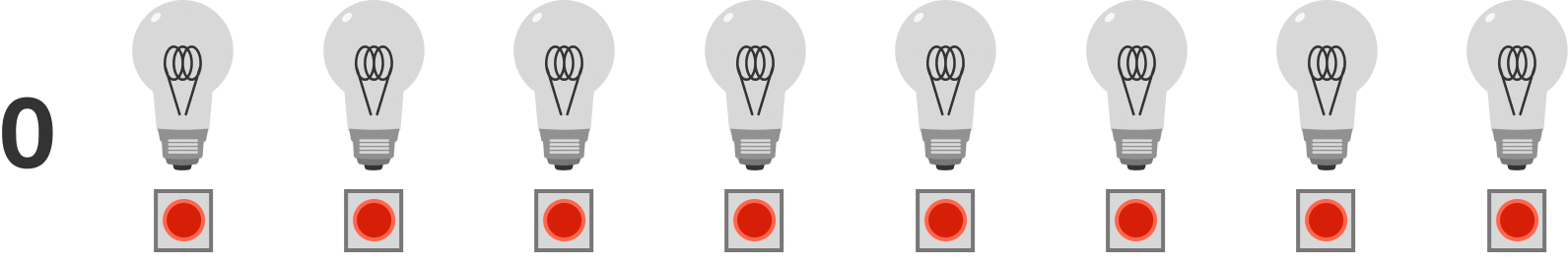Can I do it in less than 4 steps?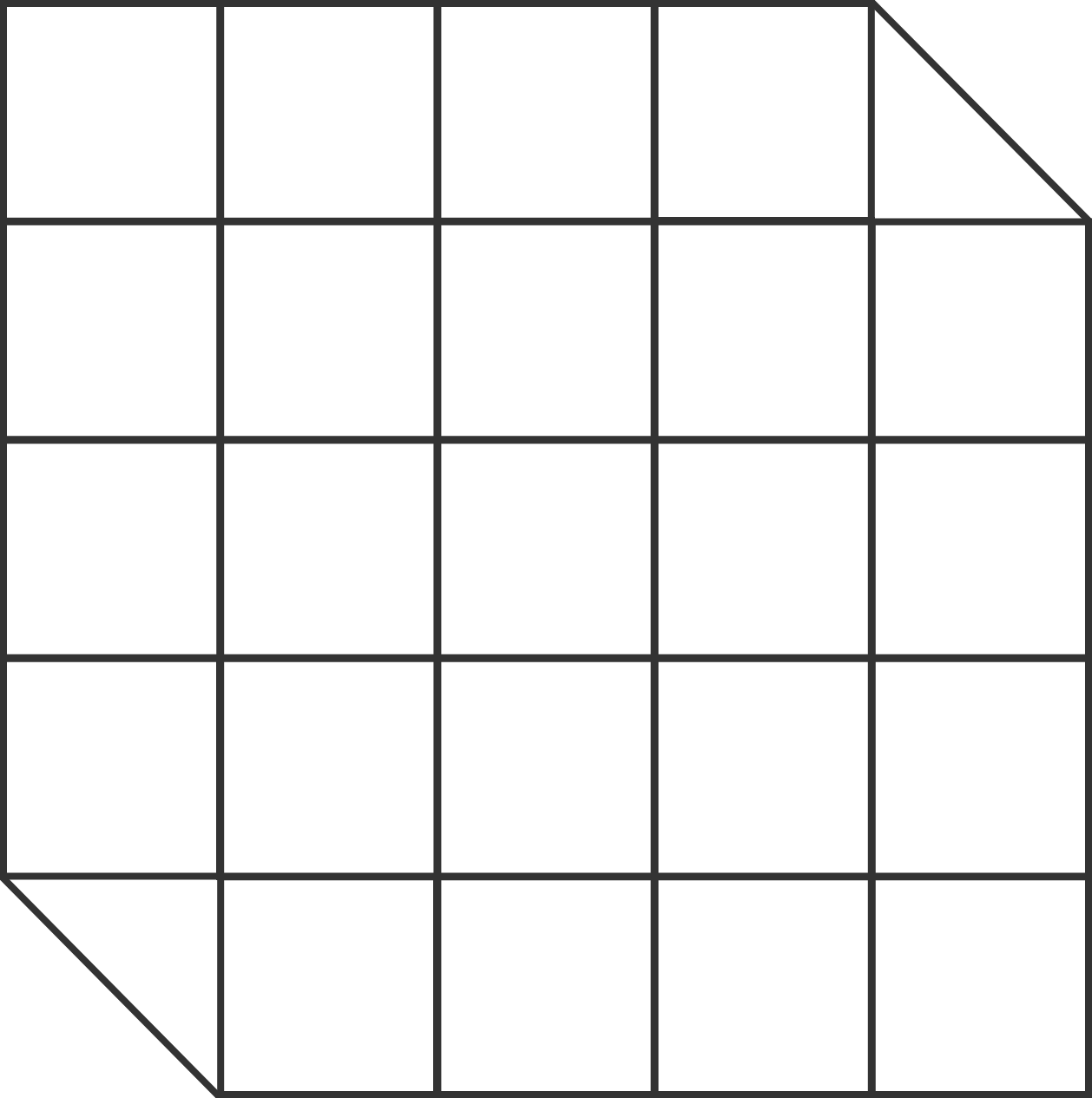Can the image to the right be cut into two equal parts with which we can form a $6\times 4$ rectangle?


Hint: You don't have to cut on the lines, and the cut can zigzag as much as you like.

×